# SAT Math Multiple Choice Question 574: Answer and Explanation

### Test Information

Question: 574

4.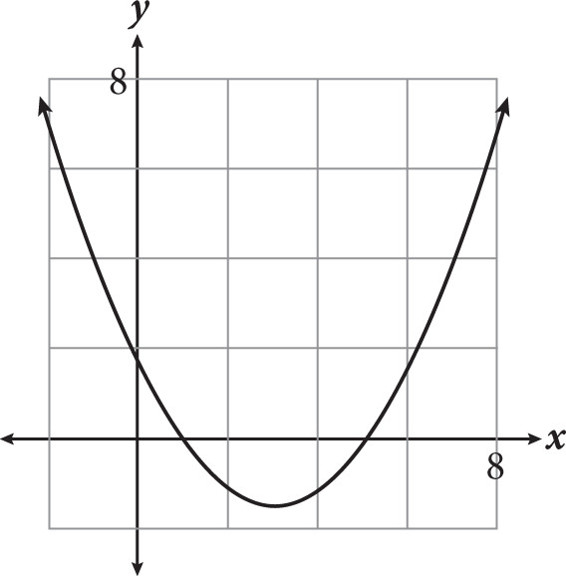The following quadratic equations are all representations of the graph shown above. Which equation could you use to find the minimum value of the function, without doing any additional work?

• A.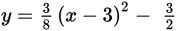• B.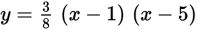• C.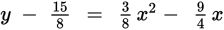• D.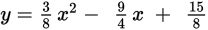Getting to the Answer: The minimum value of a quadratic function is equal to the y-value of the vertex of its graph, so vertex form, y = a(x – h)2 + k, reveals the minimum without doing any additional work. Choice (A) is the only equation written in this form and therefore must be correct. The minimum value of this function is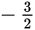.